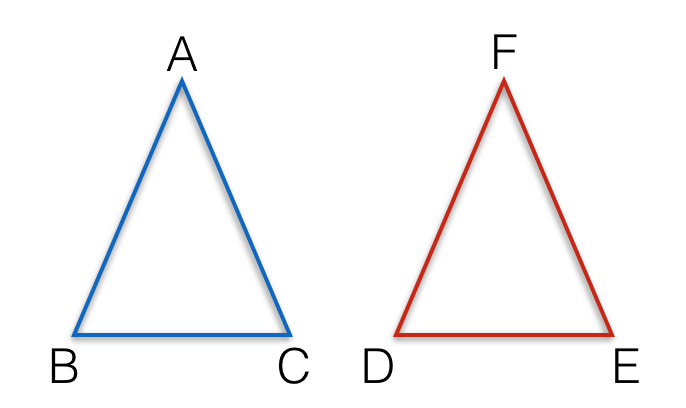# Are They Congruent?

Geometry Level 1In triangles $ABC$ and $DEF$, if we know that $AB = EF, BC = DE$ and $\angle ABC = \angle DEF$, are the triangles congruent?

×